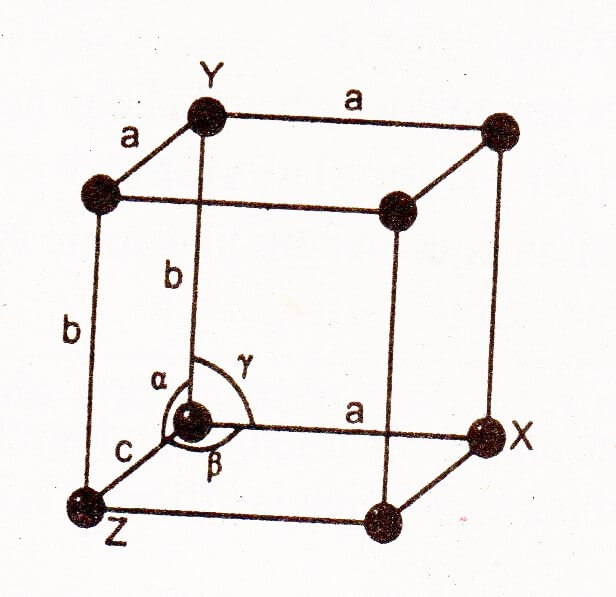A crystal lattice is defined as an array of points representing atoms, ions or molecules of a crystal, arranged at different sites in three dimensional space.

### Formation:

A crystal may contains atoms, ions or molecules. In crystalline solids, these atoms, ions or molecules are located at definite positions in space. These are represented by points in a crystal. These points are called as lattice points or lattice sites. This arrangement of points in a crystal is called crystal lattice or space lattice.

## Unit Cell Definition Chemistry:

“Unit cell is the smallest part of the crystal lattice which possesses all the characteristic feature of the space lattice, that if repeated in three dimension generates the entire lattice. As brick is the unit cell of a wall.”

### Explanation:

The stoichiometry density and spatial arrangement of the unit cell are equivalent to those of the whole crystal.

The unit cell of all different lattices are similar in having eight corners, however, they differ by the length of their edges (a, b and c) and by the angles opposite to them (α, β,γ).

α -Angle: It is the angle opposite to edge a i-e, the angle between the edges b and c.

β-Angle: It is the angle opposite to edge b i-e, the angle between the edges c and a.

γ-Angle: It is the angle opposite to edge c i-e, the angle between the edges a and b.There are seven types of unit cells known as seven basic crystal systems. The crystals of all the substances ever discovered can be described by this small set of unit cells.